# hidecan

library(hidecan)

## Input data

The hidecan package takes as input tibbles (data-frames) of GWAS and DE results or candidate genes. The input data-frames should contain some mandatory columns, depending on the type of data.

A list of example input datasets can be obtained via the get_example_data() function:

x <- get_example_data()

str(x, max.level = 1)
#> List of 3
#>  $GWAS: tibble [35,481 × 4] (S3: tbl_df/tbl/data.frame) #>$ DE  : tibble [10,671 × 7] (S3: tbl_df/tbl/data.frame)
#>  \$ CAN : tibble [32 × 6] (S3: tbl_df/tbl/data.frame)

### GWAS results

GWAS results should be provided as a tibble or data-frame, with one row per genetic marker. The data-frame should contain at least the following columns:

• chromosome: character column, giving the ID of the chromosome on which each marker is located;

• position: numeric column, the physical position along the chromosome (in base pairs - bp) of the marker;

• either score or padj: numeric column, providing either the score (i.e. -log10(p-value)) or the adjusted p-value of the marker. If a score column is provided, the padj column will be ignored. If only a padj column is provided, a score column will be constructed as -log10(padj).

Any other column present in the data-frame will be ignored. An example of valid input is shown below:

head(x[["GWAS"]])
#> # A tibble: 6 × 4
#>   id                  chromosome position score
#>   <chr>               <chr>         <dbl> <dbl>
#> 1 ST4.03ch00_45467783 ST4.03ch00 45467783 0.191
#> 2 ST4.03ch01_88589716 ST4.03ch01 88589716 1.84
#> 3 ST4.03ch02_48614228 ST4.03ch02 48614228 0.381
#> 4 ST4.03ch03_62263578 ST4.03ch03 62263578 0.661
#> 5 ST4.03ch04_72139135 ST4.03ch04 72139135 0.640
#> 6 ST4.03ch05_52040302 ST4.03ch05 52040302 0.346

### Differential expression results

DE results should be provided as a tibble or data-frame, with one row per gene. The data-frame should contain at least the following columns:

• chromosome: character column, giving the ID of the chromosome on which each gene is located;

• start and end: numeric columns, giving the starting and end position of the gene along the chromosome (in bp). These two columns will be used to calculate the position of the gene as the half-way point between the start and end of the gene.

• either score or padj: numeric column, providing either the score (i.e. -log10(p-value)) or the adjusted p-value of the gene. If a score column is provided, the padj column will be ignored. If only a padj column is provided, a score column will be constructed as -log10(padj).

• either foldChange or log2FoldChange: numeric column, giving either the fold-change or log2(fold-change) of the gene. If a log2FoldChange column is provided, the foldChange column will be ignored. If only a foldChange column is provided, a log2FoldChange will be constructed as log2(foldChange).

Any other column present in the data-frame will be ignored. An example of valid input is shown below:

head(x[["DE"]])
#> # A tibble: 6 × 7
#>   gene                 chromosome   padj log2FoldChange    start      end label
#>   <chr>                <chr>       <dbl>          <dbl>    <dbl>    <dbl> <chr>
#> 1 PGSC0003DMG400032056 ST4.03ch00  0.787       0.0114   45813195 45813526 Prote…
#> 2 PGSC0003DMG400018039 ST4.03ch01  0.630       0.00529  88623473 88627702 PhD-f…
#> 3 PGSC0003DMG400020231 ST4.03ch02  0.864       0.00362  48563271 48578978 Acety…
#> 4 PGSC0003DMG400009197 ST4.03ch03  0.530       0.0320   62256322 62258929 Phosp…
#> 5 PGSC0003DMG403025662 ST4.03ch04  0.975       0.00225  72168842 72170119 Conse…
#> 6 PGSC0003DMG400023316 ST4.03ch05 NA          -0.000726 52039916 52040326 Conse…

(Note that in the example dataset, some genes have missing values in the padj column; this corresponds to genes that have been filtered out via independent filtering in the DESeq2 package).

### Candidate genes

A list of candidate genes (e.g. genes previously found associated with a trait of interest based on literature search) can be provided as a tibble or data-frame, with one row per gene. This data-frame can also contain variants of interest (see below). The data-frame should contain at least the following columns:

• chromosome: character column, giving the ID of the chromosome on which each gene is located;

• start and end: numeric columns, giving the starting and end position of the gene along the chromosome (in bp). These two columns will be used to calculate the position of the gene as the half-way point between the start and end of the gene. For genomic variants or markers, simply set both the start and end columns to the physical position of the marker.

• name: character column, giving the name of the candidate gene that will be displayed in the HIDECAN plot. Set to NA for any subset of genes to remove their label in the plot (can help if many genes are very close, to avoid cluttering the plot).

Any other column present in the data-frame will be ignored. An example of valid input is shown below:

head(x[["CAN"]])
#> # A tibble: 6 × 6
#>   id                   chromosome    start      end name       gene_name
#>   <chr>                <chr>         <dbl>    <dbl> <chr>      <chr>
#> 1 PGSC0003DMG400003155 ST4.03ch03 46757152 46762127 4CL        4-coumarate-CoA …
#> 2 PGSC0003DMG400014223 ST4.03ch03 57466692 57469946 4CL2       4-coumarate-CoA …
#> 3 PGSC0003DMG400011189 ST4.03ch07  1001854  1006278 HQT        HQT
#> 4 PGSC0003DMG400005492 ST4.03ch05 36342746 36347409 PAL        phenylalanine am…
#> 5 PGSC0003DMG400005279 ST4.03ch05 42523943 42525912 peroxidase peroxidase
#> 6 PGSC0003DMG400007782 ST4.03ch03 38537202 38540209 PHO1A      PHO1A

## Creating a HIDECAN plot

The hidecan_plot() function creates a HIDECAN plot. It takes as input the data-frames presented above, as well as the score and log2(fold-change) thresholds used to select the significant markers and genes.

In this example, we only show markers with a score above 4, which corresponds to a p-value below $$1\times10^{-4}$$, and genes with a score above 1.3, which corresponds to a p-value of 0.05. We don’t place any threshold on the log2(fold-change) of the genes:

hidecan_plot(
gwas_list = x[["GWAS"]],          ## data-frame of GWAS results
de_list = x[["DE"]],              ## data-frame of DE results
can_list = x[["CAN"]],            ## data-frame of candidate genes
score_thr_gwas = -log10(0.0001),  ## sign. threshold for GWAS
score_thr_de = -log10(0.05),      ## sign. threshold for DE
log2fc_thr = 0                    ## log2FC threshold for DE
)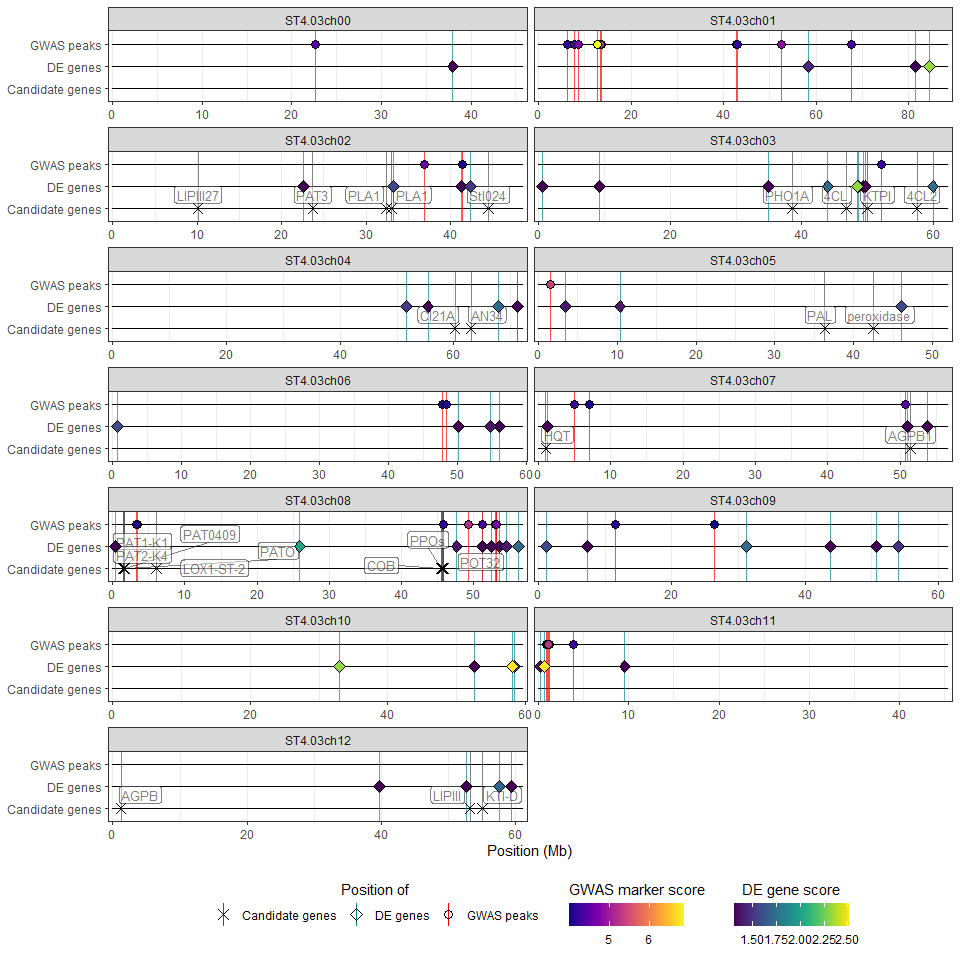Note that it is possible to provide only a subset of the possible input data, e.g. only GWAS results and a list of candidate genes:

hidecan_plot(
gwas_list = x[["GWAS"]],
can_list = x[["CAN"]],
score_thr_gwas = 4
)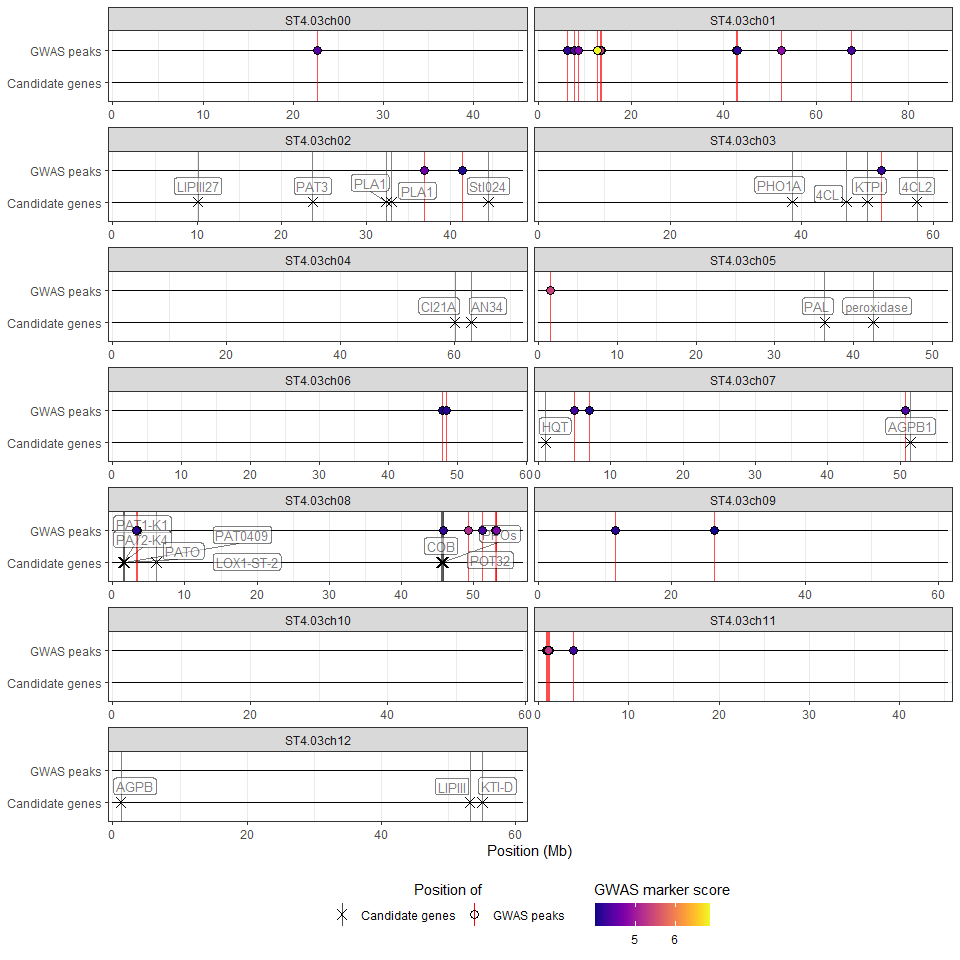## Removing empty chromosomes

By default, the HIDECAN plot shows all chromosomes present in the input data. However, it is possible that some of the chromosomes appear empty, as they do not contain any significant gene or marker, nor any candidate gene. In this case, it is possible to exclude such “empty” chromosomes from the HIDECAN plot, through the remove_empty_chrom argument.

We will demonstrate that by increasing the score threshold applied to the GWAS results, in order to get fewer significant markers. In this case, chromosomes 0, 6, 9 and 10 do not contain any significant marker of gene of interest:

## Chromosomes 0, 6, 9 and 10 are empty
hidecan_plot(
gwas_list = x[["GWAS"]],
can_list = x[["CAN"]],
score_thr_gwas = 5
)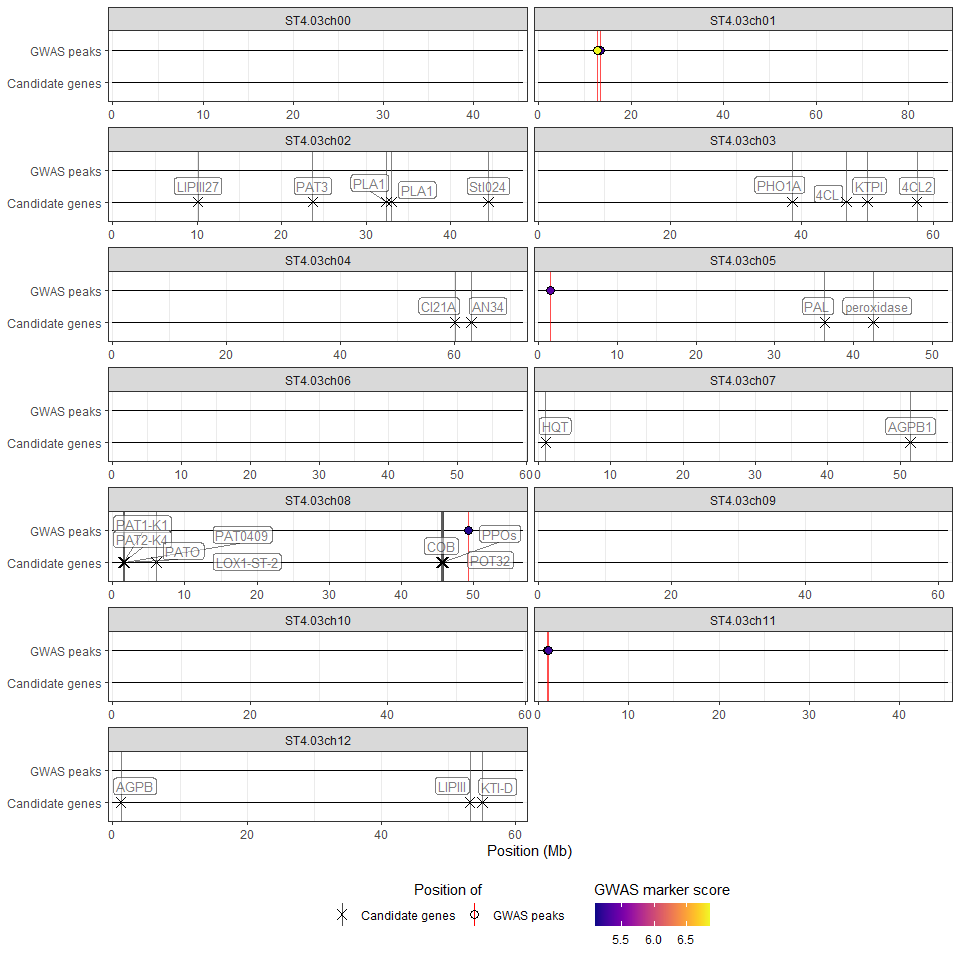By setting the remove_empty_chrom argument to TRUE, these chromosomes will be removed from the plot:

hidecan_plot(
gwas_list = x[["GWAS"]],
can_list = x[["CAN"]],
score_thr_gwas = 5,
remove_empty_chrom = TRUE
)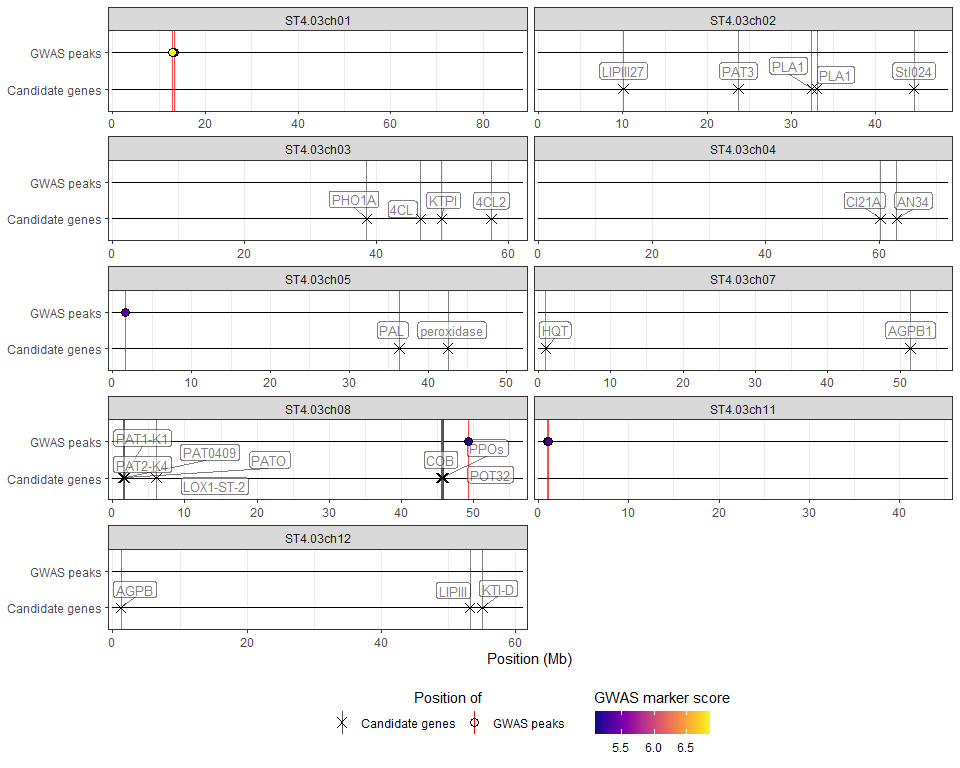## Selecting chromosomes and genomic positions

It is possible to specify which chromosomes should be represented in the HIDECAN plot, via the chroms argument. For example, with the following command we restrict the plot to chromosomes 7 and 8:

hidecan_plot(
gwas_list = x[["GWAS"]],
de_list = x[["DE"]],
can_list = x[["CAN"]],
score_thr_gwas = -log10(0.0001),
score_thr_de = -log10(0.05),
log2fc_thr = 0,
chroms = c("ST4.03ch07", "ST4.03ch08")
)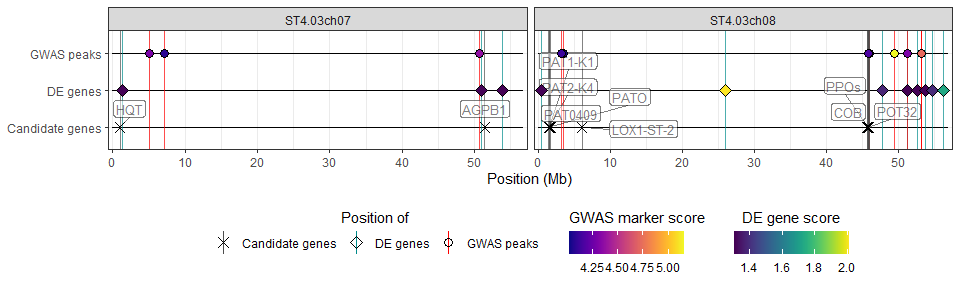We can also “zoom in” on some or all chromosomes, through the chrom_limits argument. To zoom in on all chromosomes at once, we pass to the chrom_limits argument an integer vector of length 2, which gives the lower and upper limits in bp to use. For example here, we focus on the 10-20Mb region of each chromosome:

hidecan_plot(
gwas_list = x[["GWAS"]],
de_list = x[["DE"]],
can_list = x[["CAN"]],
score_thr_gwas = -log10(0.0001),
score_thr_de = -log10(0.05),
log2fc_thr = 0,
chrom_limits = c(10e6, 20e6)
)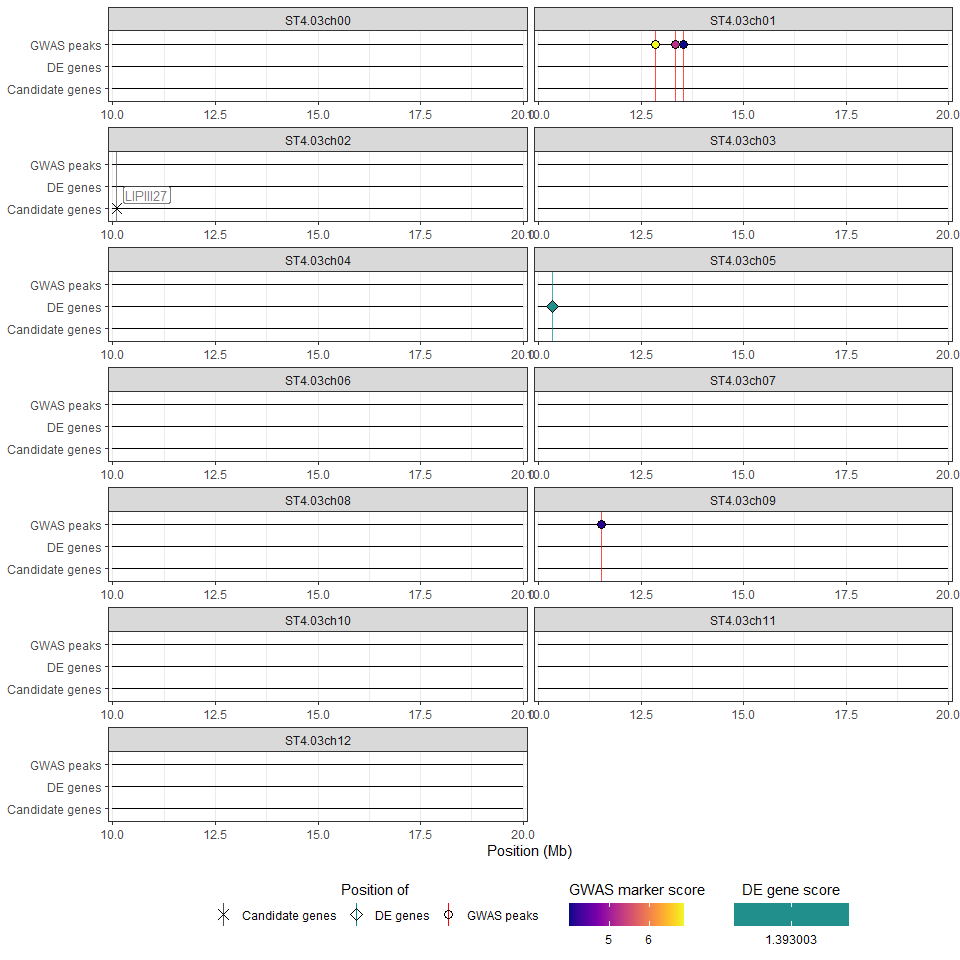Alternatively, we can apply different limits to some of the chromosomes, by passing a named list to the argument. The names of the list should match the chromosomes name, and each element should be an integer vector of length 2 giving the lower and upper limits in bp to use for the corresponding chromosome. For example, we will focus on the 10-20Mb region for chromosome 1, and the 30-40Mb region for chromosome 5, and leave all other chromosomes as is:

hidecan_plot(
gwas_list = x[["GWAS"]],
de_list = x[["DE"]],
can_list = x[["CAN"]],
score_thr_gwas = -log10(0.0001),
score_thr_de = -log10(0.05),
log2fc_thr = 0,
chrom_limits = list("ST4.03ch01" = c(10e6, 20e6),
"ST4.03ch05" = c(30e6, 40e6))
)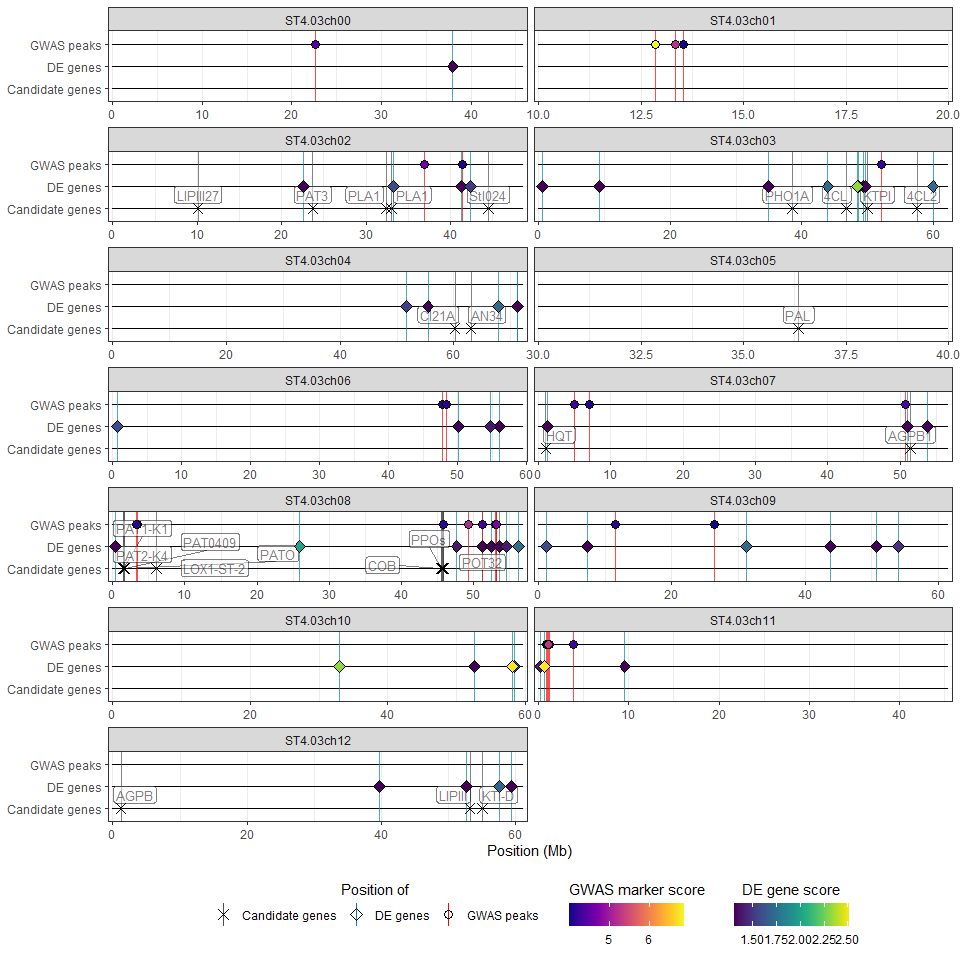The two options chroms and chrom_limits can be used together:

hidecan_plot(
gwas_list = x[["GWAS"]],
de_list = x[["DE"]],
can_list = x[["CAN"]],
score_thr_gwas = -log10(0.0001),
score_thr_de = -log10(0.05),
log2fc_thr = 0,
chroms = c("ST4.03ch07", "ST4.03ch08"),
chrom_limits = list("ST4.03ch07" = c(50e6, 55e6),
"ST4.03ch08" = c(45e6, 50e6))
)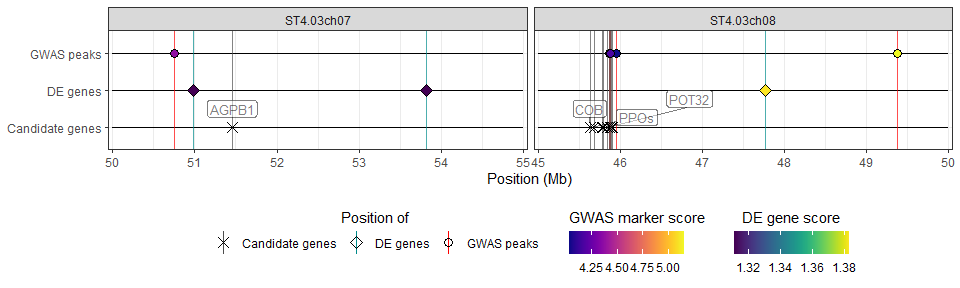## Colour genes by log2(fold-change)

By default, in a HIDECAN plot, the points representing both significant markers and DE genes are coloured according to their GWAS/DE score. However, it is possible to colour the DE genes by their log2(fold-change) value instead, by setting the colour_genes_by_score argument to FALSE:

hidecan_plot(
gwas_list = x[["GWAS"]],
de_list = x[["DE"]],
can_list = x[["CAN"]],
score_thr_gwas = -log10(0.0001),
score_thr_de = -log10(0.05),
log2fc_thr = 0,
colour_genes_by_score = FALSE
)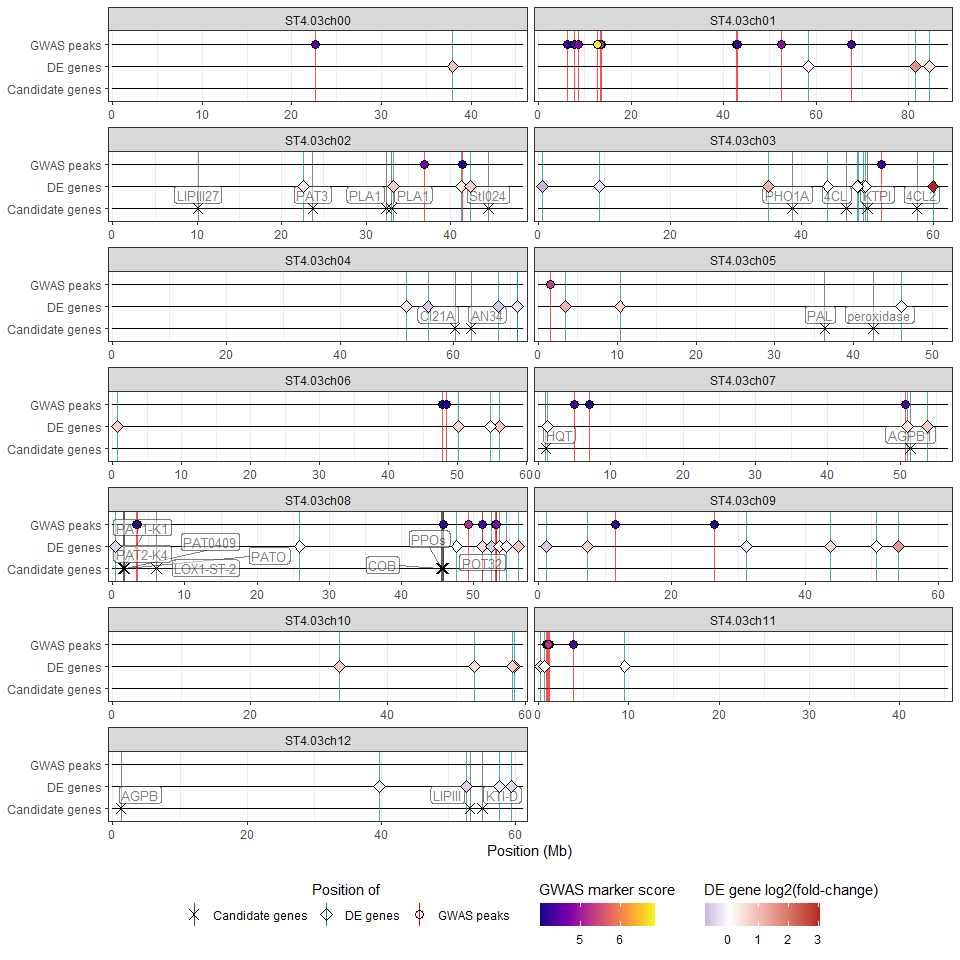Genes with a negative log2(fold-change) will be represented with a shade of blue, and genes with a positive log2(fold-change) will be represented with a shade of red.

## More than one GWAS, DE or candidate gene list

The hidecan_plot() function can take as an input lists of data-frames for GWAS results, DE results or candidate genes. This way, it is possible to visualise more than one GWAS or DE analyses at once, for example if investigating several traits at once or comparing more than two treatment groups.

For this example, we’ll focus on chromosomes 7 and 8 (only for clarity of the plot):

library(dplyr)
library(purrr)
library(stringr)

## Retaining only markers and genes on chromosomes 7 and 8
x_small <- x |>
map(~ filter(.x, str_detect(chromosome, "(07|08)")))

We’ll create a second data-frame of GWAS results by shuffling the marker scores in the example dataset:

## Creating a second GWAS result tibble by shuffling
## the marker scores from the original data
gwas_1 <- x_small[["GWAS"]]
gwas_2 <- gwas_1 |>
mutate(score = sample(score))

We can pass both GWAS results data-frames to the hidecan_plot() function as a list:

hidecan_plot(
gwas_list = list(gwas_1, gwas_2),
score_thr_gwas = -log10(0.0001),
score_thr_de = -log10(0.05),
log2fc_thr = 0
)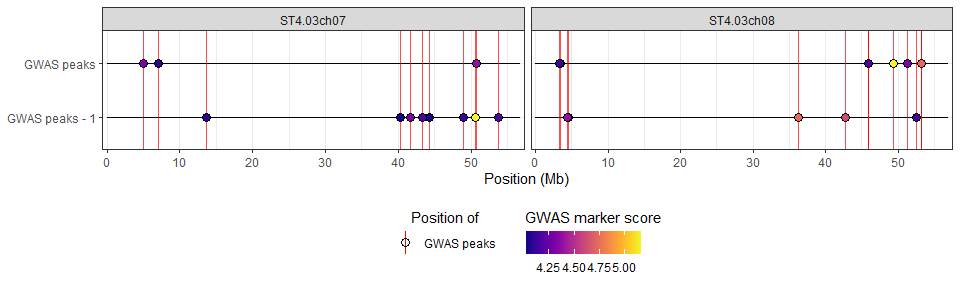By default, the two GWAS tracks will be given unique y-axis labels, as can be seen above. It is possible to customise this by naming the elements in the input list:

hidecan_plot(
gwas_list = list("Trait 1" = gwas_1,
"Trait 2" = gwas_2),
score_thr_gwas = -log10(0.0001),
score_thr_de = -log10(0.05),
log2fc_thr = 0
)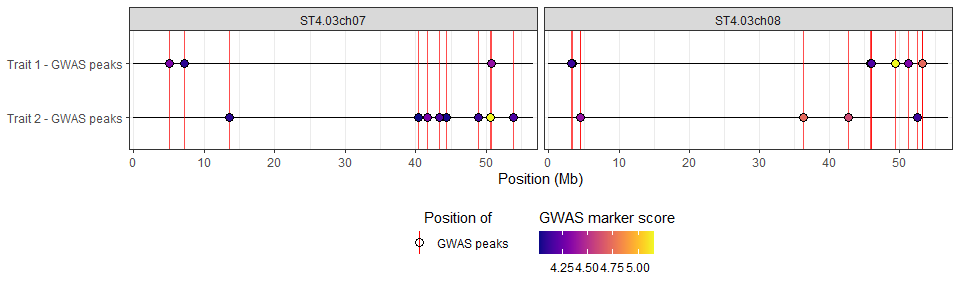## Defining chromosomes length

By default, the hidecan_plot() function calculates the length of the different chromosomes based on the input data, by looking at the maximum position of genes and markers on each chromosome. However, it is also possible to pass on a tibble of chromosome length (in bp) through the chrom_length argument.

library(tibble)

## Chromosomes length as recorded in Ensembl Plants
potato_chrom_length <- c(
ST4.03ch00 = 45813526,
ST4.03ch01 = 88663952,
ST4.03ch02 = 48614681,
ST4.03ch03 = 62190286,
ST4.03ch04 = 72208621,
ST4.03ch05 = 52070158,
ST4.03ch06 = 59532096,
ST4.03ch07 = 56760843,
ST4.03ch08 = 56938457,
ST4.03ch09 = 61540751,
ST4.03ch10 = 59756223,
ST4.03ch11 = 45475667,
ST4.03ch12 = 61165649
) |>
## turn a named vector into a tibble
enframe(name = "chromosome",
value = "length")

#> # A tibble: 6 × 2
#>   chromosome   length
#>   <chr>         <dbl>
#> 1 ST4.03ch00 45813526
#> 2 ST4.03ch01 88663952
#> 3 ST4.03ch02 48614681
#> 4 ST4.03ch03 62190286
#> 5 ST4.03ch04 72208621
#> 6 ST4.03ch05 52070158
hidecan_plot(
gwas_list = x[["GWAS"]],
de_list = x[["DE"]],
can_list = x[["CAN"]],
score_thr_gwas = -log10(0.0001),
score_thr_de = -log10(0.05),
log2fc_thr = 0,
chrom_length = potato_chrom_length
)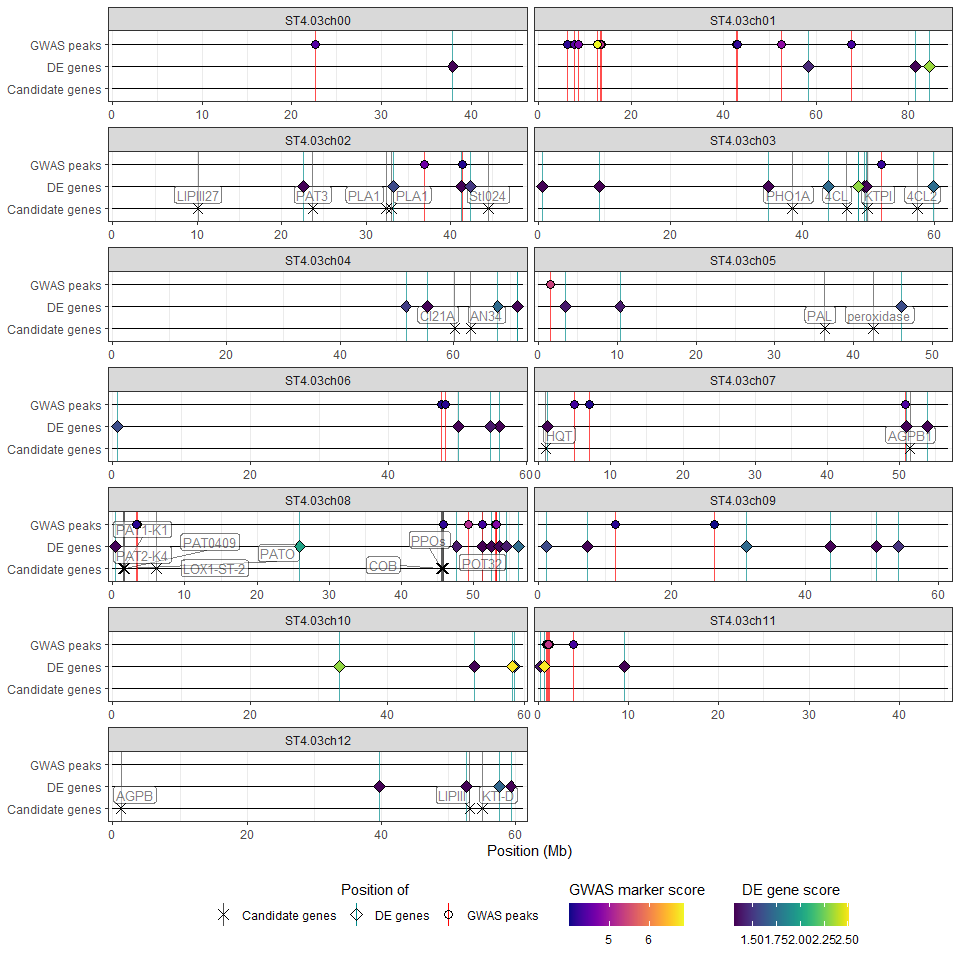Note that in this case we can’t really see the difference with the computed chromosome length values.

## Controlling the plot properties

The hidecan_plot() function offers several arguments to control different aspects of the HIDECAN plot. For example, it is possible to specify the number of rows or columns the plot should have, through the n_rows and n_cols arguments. Note that only one of these arguments will be considered (n_rows takes precedence):

## Specifying the number of rows
hidecan_plot(
gwas_list = x[["GWAS"]],
de_list = x[["DE"]],
can_list = x[["CAN"]],
score_thr_gwas = -log10(0.0001),
score_thr_de = -log10(0.05),
log2fc_thr = 0,
n_rows = 3
)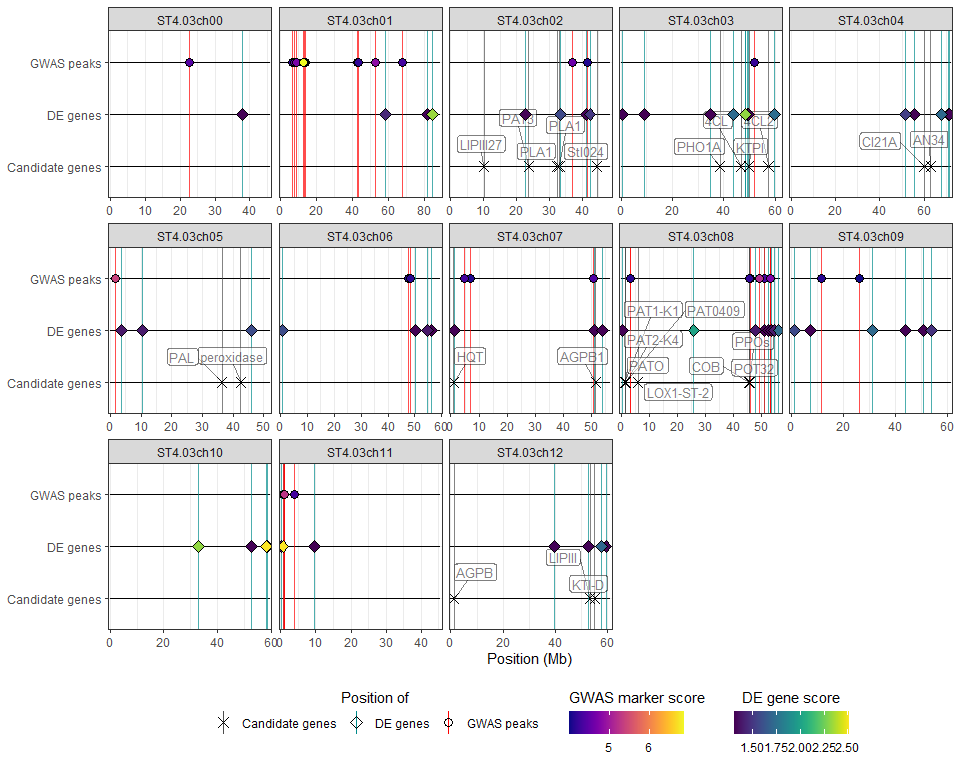## Specifying the number of columns
hidecan_plot(
gwas_list = x[["GWAS"]],
de_list = x[["DE"]],
can_list = x[["CAN"]],
score_thr_gwas = -log10(0.0001),
score_thr_de = -log10(0.005),
log2fc_thr = 0,
n_cols = 3
)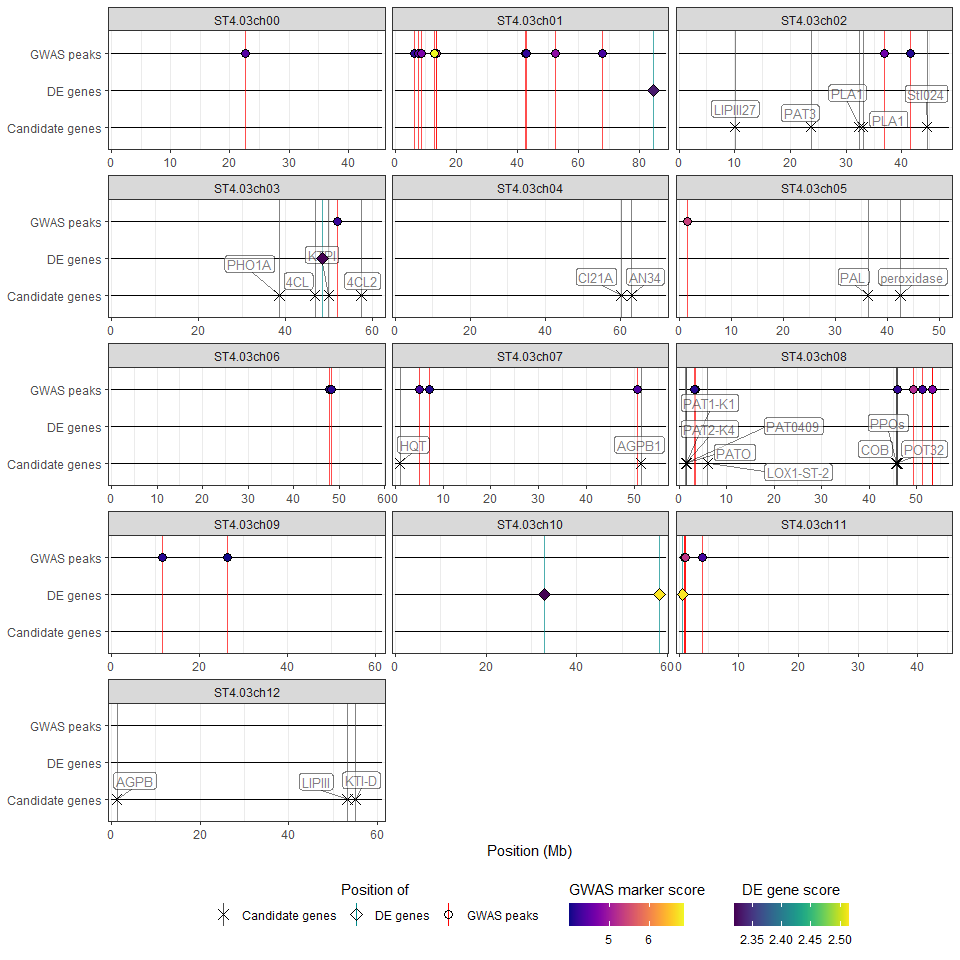In addition, it is possible to:

• add a title and subtitle to the plot (title and subtitle arguments);

• control the position of the legend (legend_position argument);

• control the size of the points (point_size argument);

• control the size of the labels and padding around the text for the candidate genes labels (label_size and label_padding arguments).

## Viewport error

If you are working on RStudio, you may encounter the following error:

#> Error in grid.Call(C_convert, x, as.integer(whatfrom), as.integer(whatto), : Viewport has zero dimension(s)

This is caused by the plotting window being too small. Try increasing the size of the plotting window in the RStudio console. Alternatively, you can save the plot into an R object, then use ggplot2::ggsave() to save it into a file:

p <- hidecan_plot(
gwas_list = x[["GWAS"]],
de_list = x[["DE"]],
can_list = x[["CAN"]],
score_thr_gwas = -log10(0.0001),
score_thr_de = -log10(0.05),
log2fc_thr = 0,
label_size = 2
)

ggplot2::ggsave("hidecan_plot.pdf", p, width = 10, height = 10)